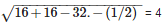# Conic Sections - Online Test

Q1. If the line 2x – y +  = 0 is a diameter of the circle  then  =
Explaination / Solution:

Equation of circle is

Applying completing the square method

Comparing the above equation with   we get center as (-3,3) and radius as    .

As centre of the circlre lies on diameter , it will satisfy the equation of diameter, so on putting (-3,3) in equation of diameter we get

=>

=>

Q2. Length of common chord of the circles and  is
Explaination / Solution: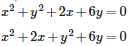Applying the completing the square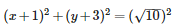Comparing the above equation with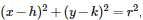we get its center as (-1,-3) and radius (r1)=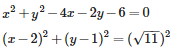Comparing the above equation with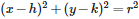we get its center as (2,1) and radius (r2)=

So distance(d) between the centers isunits
After applying general formula of length of common chord as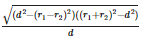and putting the values of r1, r2 and d we get length as
.

Q3. The circles  and
Explaination / Solution:

Circle touches externally if distance between the centers is equal to the sum of the radii .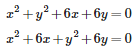After applying completing the square, we get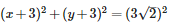so the center is (-3,-3) and radius is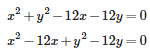After applying completing the square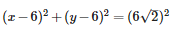so the center is (6,6) and radius is
Distance between centres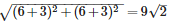and sum of radii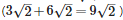are equal.

Hence the circle touches externally.

Q4.

The equation  represents

Explaination / Solution:

The above circle can be written as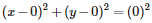Here the center is (0,0) and radius is also 0 units.

So it is a degenerate circle as  degenerate circle is a circle( a point) where radius is zero units.

Q5.

The equation represents

Explaination / Solution:

The general equation of the circle is x2+y2-2gh-2fy+c = 0. Sice the given equations satisfies the general equation, it represents the equation of the circle.

Q6. Circumcentre of the triangle, whose vertices are (0, 0), (6, 0) and (0, 4) is
Explaination / Solution:

circumcentre of a right angled triangle ABC right angled at A is  as circumcentre of right angled triangle lies on the mid pont of the hypotenuse.

so mid point of BC=(,) i.e.(3,2)

Q7. If (x-a)+ (y-b)= c2 represents a circle, then
Explaination / Solution:

(x-a)+ (y-b)= c2  here (a,b) is center and c is the radius

and radius cannot be zero because if radius is zero it will become a point or degenerate circle so c0.

Q8. Two perpendicular tangents to the circle  meet at P. The locus of P is
Explaination / Solution:

locus of P is a circle with centre at origin and radius .This is known as the director circle of the circle
Q9. The number of tangents to the circle which pass through the point ( 3, - 2), is
Explaination / Solution: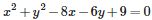After completing the square,we get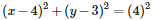so center is (4,3) and radius is 4.

Distance between center and given point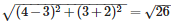which is greater than 4.

hence point lies outside the circle .

Since point lies outside of the circle there will be 2 tangents since two tangents can be drawn from external point to a circle.

Q10. The length of the chord joining the point ( 4 cos , 4 sin ) and 4 ( cos(+), 4 sin( + )) of the circle  is
Explaination / Solution:

using distance formulae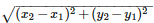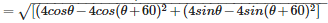on simplifying we get,